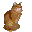Puzzle n°19 (*,***) - Somalis ad infinitum...The decimal representation of a fraction may be written with a finite number of digits (as for example 3/4 = 0,75)
or with a recurring set of digits without an end (as for example 5/37 = 0,135 135 135 135...)

The question here is to find the fraction with the smallest numerator such that its decimal representation be :

0,SOMALISOMALISOMALISOMALI... without an end

where each letter of SOMALI represents a number between 1 and 9 (no zero),
two different digits being represented by different letters
and two different letters always coding two different digits.

Recall : in a fraction, such as 6/13 for example, the numerator is 6 and the denominator is 13.
Here, you are asked to find the smallest numerator possible.

Note : the first digit before the comma is a zero,
not to be confused with the letter "O" of SOMALI which represents a digit different than 0.Clue : Difficult to find a smaller numerator...

. Once you have found the answer, try the same exercise with abyssinians :

0,ABYSSINIANABYSSINIANABYSSINIANABYSSINIAN... without an end

(each letter A,B,Y,S,I,N represents a different digit, each between 1 and 9.
Beware, the same digit must appear every time the same letter appears)

Is this a proof that abyssinians are really more complicated than somalis ???...
(not to mention that the puzzle is more complex in English than in French where ABYSSIN has 7 letters, not 10)

If you think this is too hard (you will definitely need a few arithmetics principles and a computer...),
try the abridged version :

0,ABYABYABYABYABY...

Clue : look a bit higher...